vhdl7我学习得第一个fpga项目——倒计时显示

10 篇文章 3 订阅

1.功能：
1）开关控制计数器工作。

2.思路：
1)两个分频器，一个给1s钟的倒计时计数，一个用于驱动数码管。
2)一个计数开关，控制倒计时开始
3）数码管译码电路。
3.设计：
1）首先，从顶层把几个模块定义一下，相互之间接下线，有分频器，计数器，显示模块。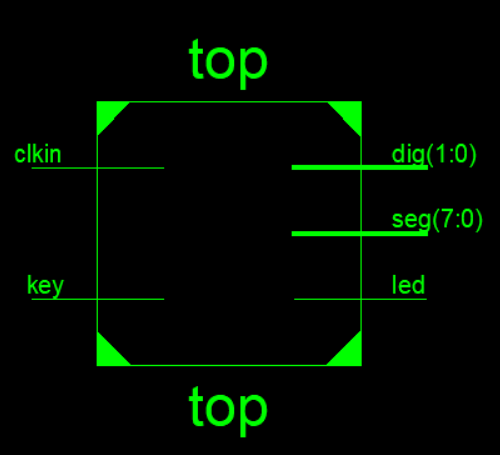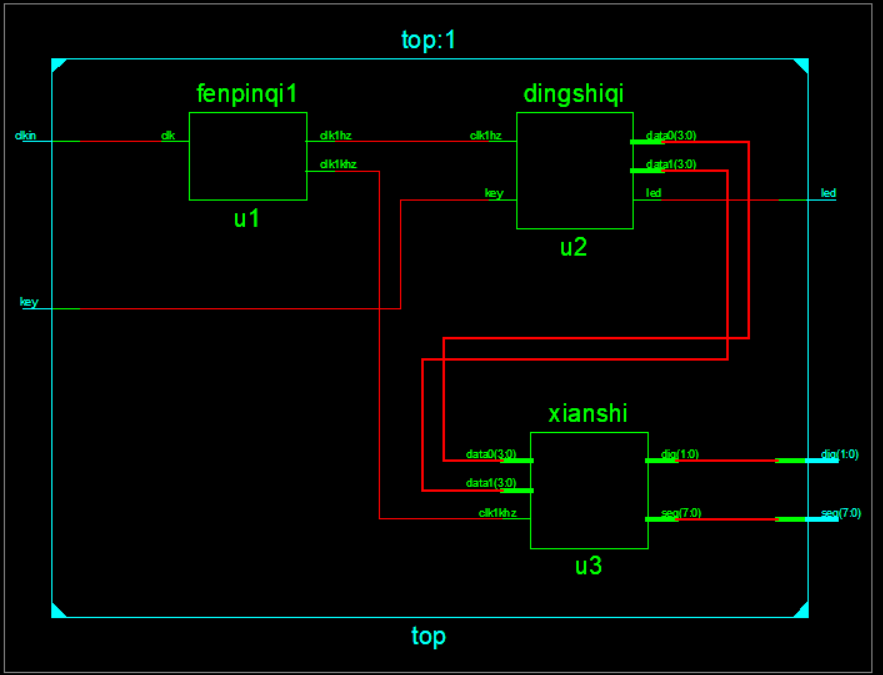通过这个电路图可以很清楚的看到倒计时计数器内部的结构。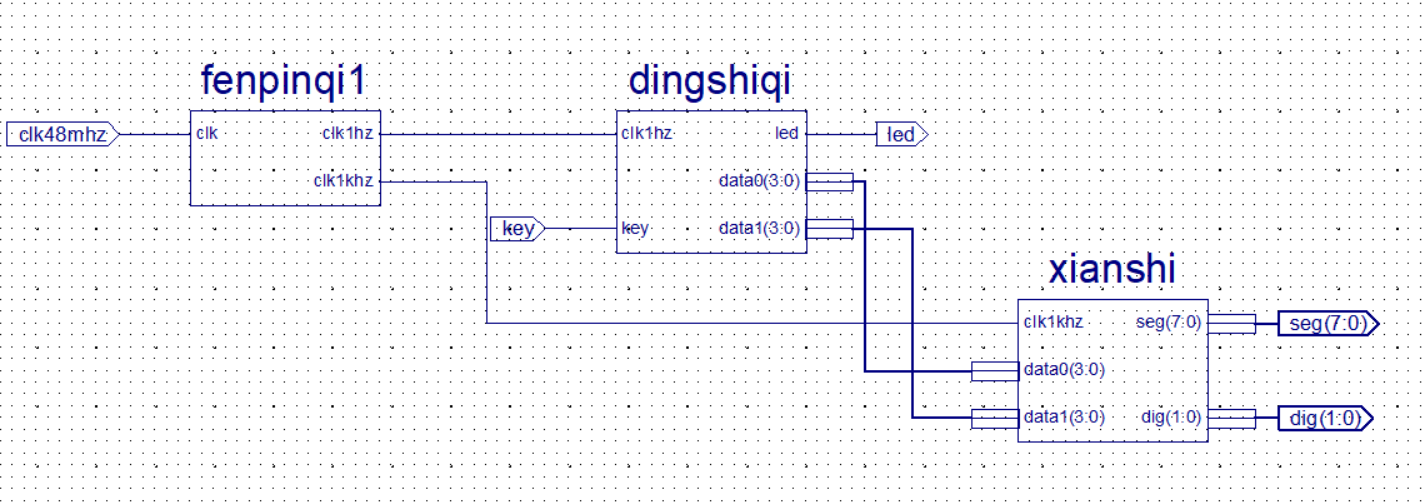3.编写子模块
1）分频器：
1.1这个之前讲过。这次犯的错误是把端口放在process中直接not了，这样会报错，说要用inout。那我直接定义signal就好了。
1.2if语句一定是出现在process中的
1.3变量只可以在当前的process中使用，只有signal才可以全局。signa定义不要加in/out！。
1.4最后记得把signal赋值给相应的port

library IEEE;
use IEEE.STD_LOGIC_1164.ALL;
--ujs-lili
entity fenpinqi1 is
Port ( clk : in  STD_LOGIC;
clk1hz : out  STD_LOGIC;
clk1khz : out  STD_LOGIC);
end fenpinqi1;
architecture Behavioral of fenpinqi1 is
signal clk_1hz : STD_LOGIC;
signal clk_1khz :  STD_LOGIC;
begin
process(clk)
variable q:integer range 1 to 2400000:=1;--48mhz,一个周期1/48m，1hz，一个周期1/1，那么1/1/（1/48m）/2，得到半个周期中clk1hz的次数2400000,因为程序中用not得到上升沿。
variable p:integer range 1 to 24000:=1;--1khz时间快。
begin
if q = 2400000 then
q:=1;
clk_1hz <= not clk_1hz;
else
q:=q+1;
end if;

if q = 24000 then
p:=1;
clk_1khz <= not clk_1khz;
else
p:=p+1;
end if;
end process;
clk1hz <=  clk_1hz;
clk1khz <= clk_1khz;
end Behavioral;

2）设计定时器

library IEEE;
use IEEE.STD_LOGIC_1164.ALL;
use IEEE.STD_LOGIC_arith.ALL;
use IEEE.STD_LOGIC_unsigned.ALL;
--ujs_lili
entity dingshiqi is
Port ( clk1hz : in  STD_LOGIC;
key : in  STD_LOGIC;
led : out STD_LOGIC;
data0 : out  STD_LOGIC_VECTOR (3 downto 0);
data1 : out  STD_LOGIC_VECTOR (3 downto 0));
end dingshiqi;
architecture Behavioral of dingshiqi is
signal cnt0: STD_LOGIC_VECTOR (3 downto 0);
signal cnt1: STD_LOGIC_VECTOR (3 downto 0);
begin
process(clk1hz,key)
begin
if key='1' then
cnt1<="0001";
cnt0<="0110";--这里用的bcd码，便于显示
led<='1';
else
led<='0';--表示开关作用
if clk1hz'event and clk1hz='1' then --1s到
if cnt0/=0 then --如果个位不等于0，个位就可以自减，如果个位等于0，这时候就要看了如果十位为0，那么计数结束，如果十位不为0，那么置十位为0
cnt0<=cnt0-1;
else
if cnt1/=0 then--如果10位不等于0，也就是1，那么就剩下9,8,7,...
cnt0<="1001";cnt1<=cnt1-1;
else--如果十位为0，计数结束，因为if是顺序执行的
cnt0<="0000";cnt1<="0000";led<='1';
end if;
end if;
end if;
end if;
end process;
data0<=cnt0;
data1<=cnt1;
end Behavioral;

3）显示模块

4）仿真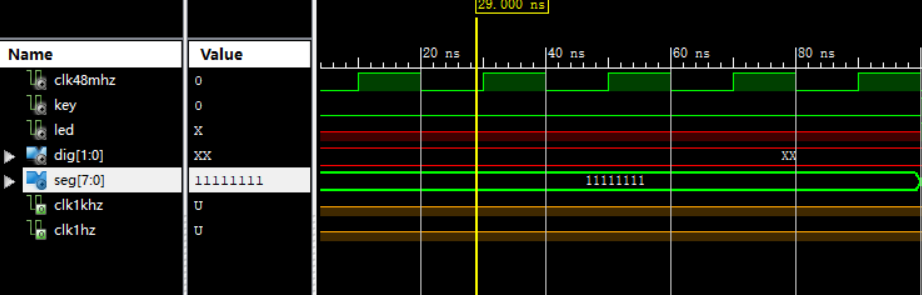2）seg没有变化
3）分频电路有问题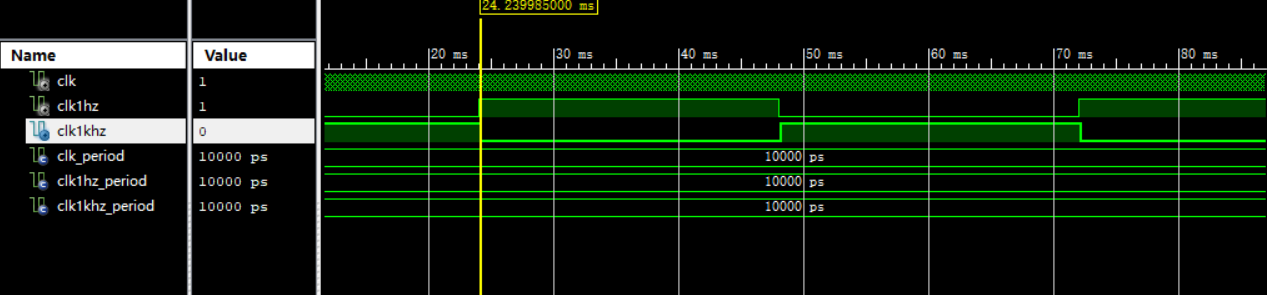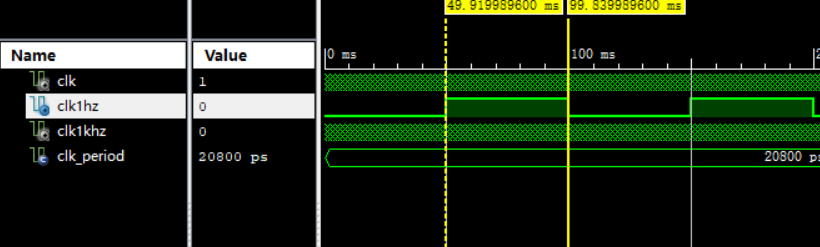library IEEE;
use IEEE.STD_LOGIC_1164.ALL;
--ujs-lili
entity ceshifenpin is
Port ( clk : in  STD_LOGIC;
clk1hz : out  STD_LOGIC;
clk1khz : out  STD_LOGIC);
end ceshifenpin;
architecture Behavioral of ceshifenpin is
signal clk_1hz : STD_LOGIC:='0';
signal clk_1khz :  STD_LOGIC:='0';
begin
process(clk)
variable q:integer range 1 to 2400000:=1;--48mhz,一个周期1/48m，1hz，一个周期1/1，那么1/1/（1/48m）/2，得到半个周期中clk1hz的次数2400000,因为程序中用not得到上升沿。
variable p:integer range 1 to 24000:=1;--1khz时间快。
begin
if clk'event and clk='1' then
if q = 2400000 then
q:=1;
clk_1hz <= not clk_1hz;
else
q:=q+1;
end if;
if p = 24000 then--这个地方没有改，应该时p！
p:=1;
clk_1khz <= not clk_1khz;
else
p:=p+1;
end if;
end if;
end process;
clk1hz <=  clk_1hz;
clk1khz <= clk_1khz;
end Behavioral;

LIBRARY ieee;
USE ieee.std_logic_1164.ALL;
--ujs-lili
ENTITY ceshifenpin_tb IS
END ceshifenpin_tb;
ARCHITECTURE behavior OF ceshifenpin_tb IS
-- Component Declaration for the Unit Under Test (UUT)
COMPONENT ceshifenpin
PORT(
clk : IN  std_logic;
clk1hz : OUT  std_logic;
clk1khz : OUT  std_logic
);
END COMPONENT;
--Inputs
signal clk : std_logic := '0';
--Outputs
signal clk1hz : std_logic;
signal clk1khz : std_logic;
-- Clock period definitions
constant clk_period : time := 20.8 ns;--10ns是10的-8
BEGIN
-- Instantiate the Unit Under Test (UUT)
uut: ceshifenpin PORT MAP (
clk => clk,
clk1hz => clk1hz,
clk1khz => clk1khz
);
-- Clock process definitions
clk_process :process
begin
clk <= '0';
wait for clk_period/2;
clk <= '1';
wait for clk_period/2;
end process;
END;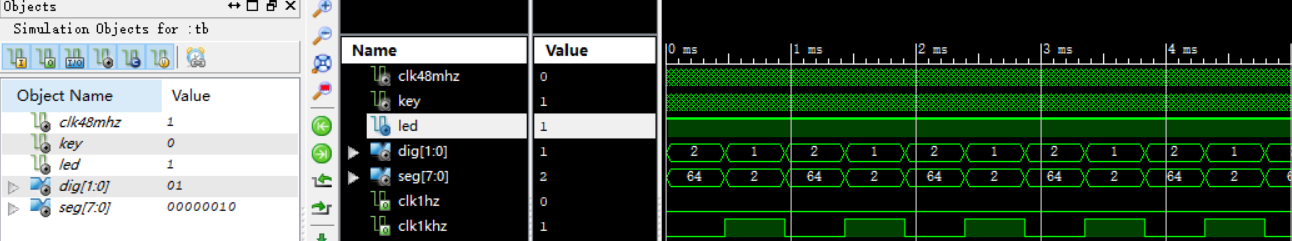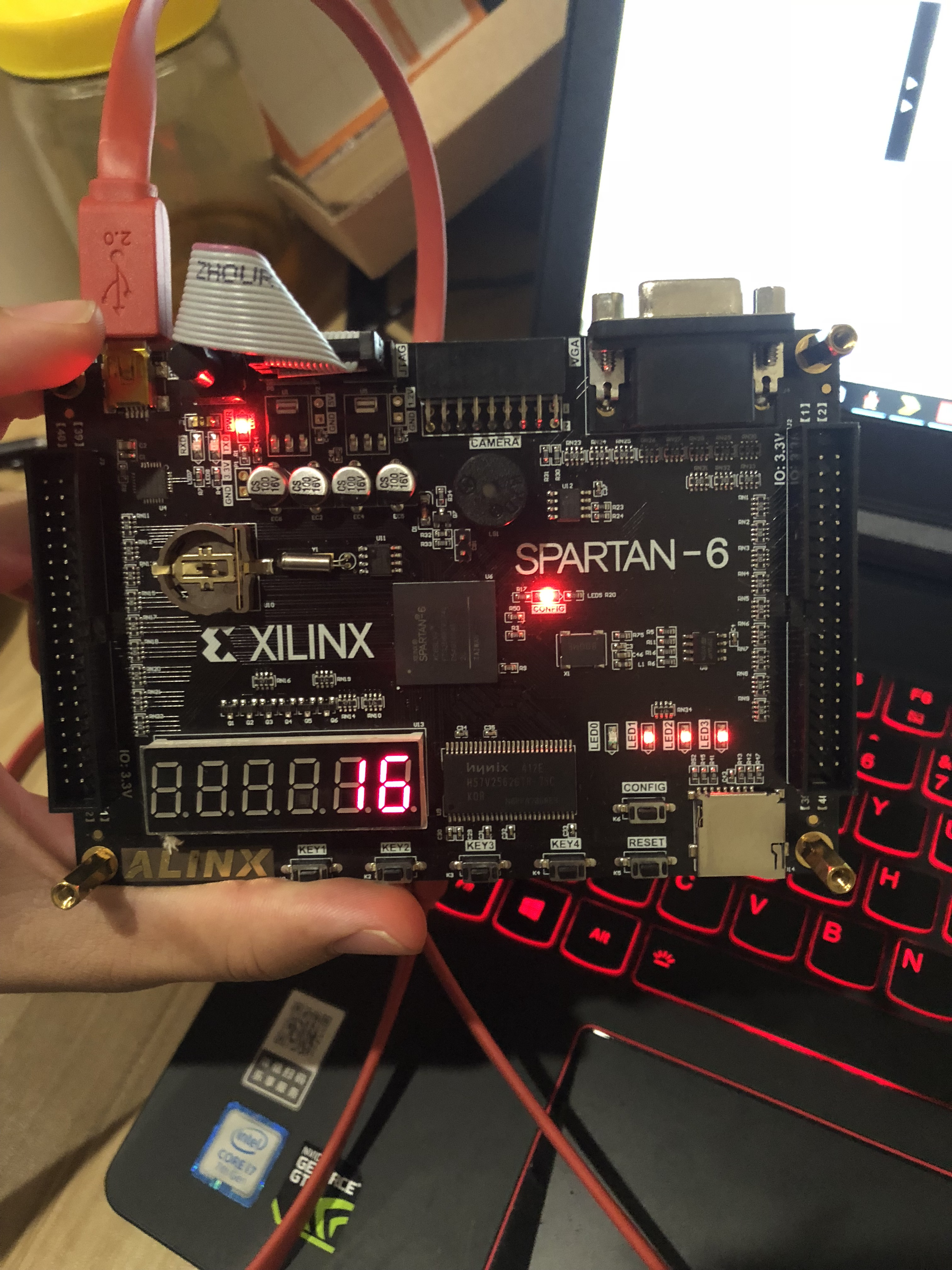6.总结

06-227910

06-13421
12-081万+
02-233710
02-221292
02-261万+
09-151978
10-181974
07-14
03-192304¥2 ¥4 ¥6 ¥10 ¥20余额支付 (余额：-- )扫码支付获取中扫码支付点击重新获取扫码支付1.余额是钱包充值的虚拟货币，按照1:1的比例进行支付金额的抵扣。
2.余额无法直接购买下载，可以购买VIP、C币套餐、付费专栏及课程。余额充值